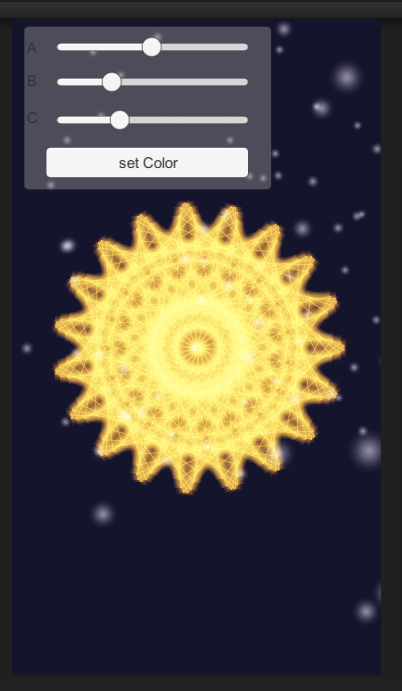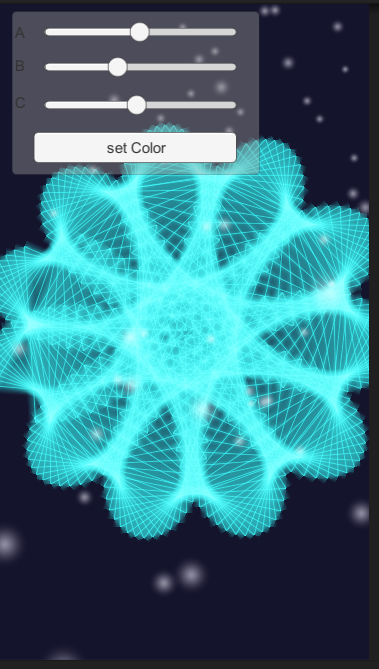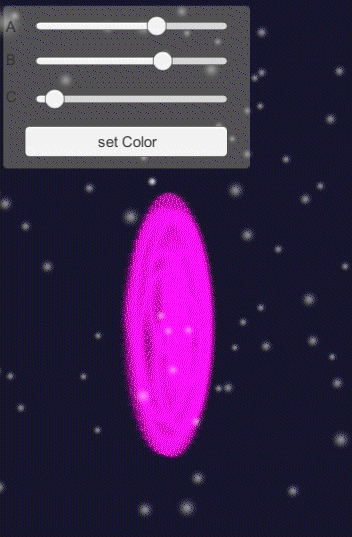# unity3d画出漂亮的玫瑰图案函数。

2018/09/04 06:40

x=(a+b)cosθ-acos[(a+b)θ/b];
y=(a+b)sinθ-asin[(a+b)θ/b];

UNITY3D具体的代码是：

``````for (var i=0; i<numberOfPoints; i++) {
theta = i * Mathf.PI / 180;
linePoints [i] = new Vector3 ((a + b) * Mathf.Cos(1*theta) -a*Mathf.Cos((a +b) * theta / b), (a +b) * Mathf.Sin (1*theta)-a* Mathf.Sin ((a +b) * theta / b), 1.0);

}``````

``````var rotateSpeed = 10.0;

function Update () {
transform.Rotate(Vector3.up * Time.deltaTime * rotateSpeed);
}``````

a、b的值可以用slider等UI控件来解决。

``````    btn=gameObject.Find("Canvas/Panel/Button").GetComponent(UI.Button);

sca=gameObject.Find("Canvas/Panel/Slidera").GetComponent(UI.Slider);

scb=gameObject.Find("Canvas/Panel/Sliderb").GetComponent(UI.Slider);

scc=gameObject.Find("Canvas/Panel/Sliderc").GetComponent(UI.Slider);

``````	function Slidera (temp:float)
{
a=  temp/100;
LineSetup (true);
}
function Sliderb (temp:float)
{
b= temp/1000;
LineSetup (true);
}``````

``````	function Sliderc (temp:float)
{
var gb= GameObject.Find("Main Camera");
gb.transform.position=new Vector3( gb.transform.position.x, gb.transform.position.y, temp);
}``````3
6 收藏

### 作者的其它热门文章2019/08/30 15:52

1 评论
6 收藏
3